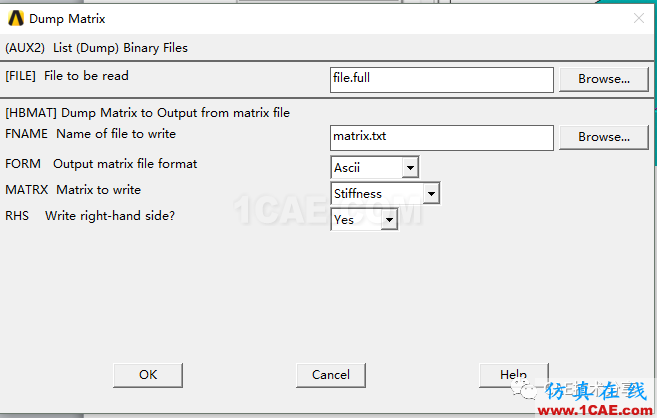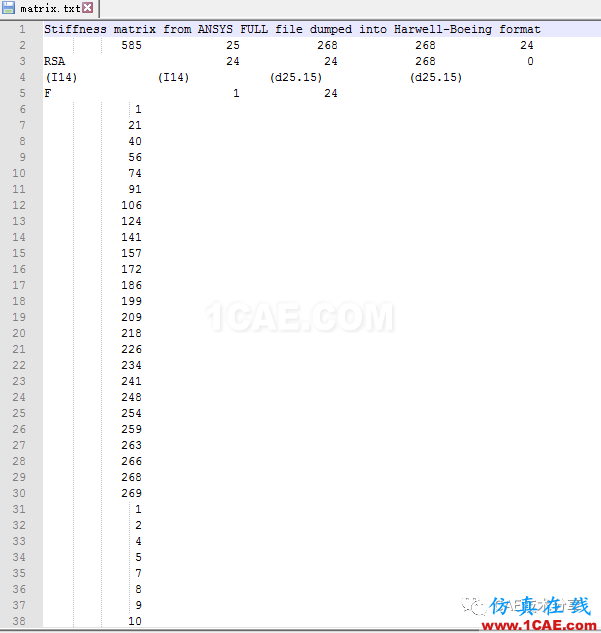# ANSYS刚度矩阵的提取与解析(python解析）

2017-09-15  by:CAE仿真在线  来源:互联网#!user/bin/python

#-* -coding:UTF-8 -*-

#打开矩阵文件

inpfile = open("E:\CAE_Tech\process_files\232Files\matrix.txt")

#读取所有内容

inpfile.close()

#打开输出文件

outfile = open("E:\CAE_Tech\process_files\232Files\outmatrix.txt",’w’)

#解析矩阵文件的第二行

row_second = lines.split()

#得到矩阵的总行数(扣除头文件),此次实例中为585

Tol_content = int(row_second)

#得到列指针的总行数

Col_tol = int(row_second)

#得到矩阵元素总数

Row_tol = int(row_second)

#得到右端项的行数

Rhs_tol = int(row_second)

#初始化矩阵每列非零元素的起始行数

Star_row_num =  * Col_tol

#获取行起始数组

for i in range (Col_tol):

temp = lines[i+5].split()

Star_row_num[i] = int(temp)

for i in range (Rhs_tol):

temp_len = Star_row_num[i+1] - Star_row_num[i]

temp_len1 = Star_row_num - Star_row_num

temp_len2 = Star_row_num[i] - Star_row_num

row_start_num = 5 + Col_tol + temp_len2

Each_col_num = i + 1

Each_col_num_str = str(Each_col_num)

for j in range (temp_len):

Each_row_num_str = lines[row_start_num + j].split()

Each_row_num = int(Each_row_num_str)

Each_row_num_str = str(Each_row_num)

Each_ele_num = Row_tol +5 + Col_tol + temp_len2 + j

Each_ele_str = lines[Each_ele_num].split()

Each_ele_value = Each_ele_str

#得到每一行应该输出的列号,行号以及元素值

Each_row_list = Each_row_num_str,Each_col_num_str,Each_ele_value,'\n'

a = ' '

Each_line = a.join(Each_row_list)

outfile.write(Each_line)

#输出右端项

for i in range (Rhs_tol):

Rhs_num = Tol_content + 5 -Rhs_tol +i

Rhs_each_row = lines[Rhs_num]

#去掉字符串前面的空格

Rhs_each_row = Rhs_each_row.lstrip()

outfile.write(Rhs_each_row)

outfile.close()• 客服在线请直接联系我们的客服，您也可以通过下面的方式进行在线报名，我们会及时给您回复电话，谢谢！